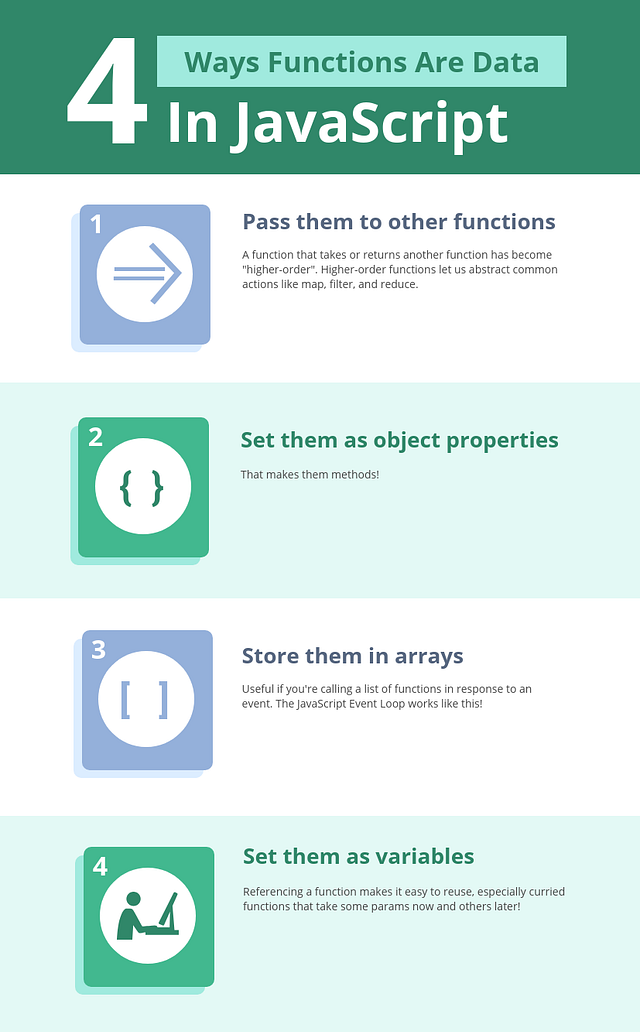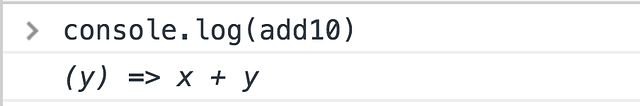# An introduction to Higher-Ordaer Functions in JavaScript

• 2019-03-12 12:36 AM
• 48

Use functions as data, and unlock some powerful patterns.

### Higher-Order Functions

A function that accepts and/or returns another function is called a higher-order function.

It’s higher-order because instead of strings, numbers, or booleans, it goes higher to operate on functions. Pretty meta.

With functions in JavaScript, you can

• Store them as variables
• Use them in arrays
• Assign them as object properties (methods)
• Pass them as arguments
• Return them from other functions

Like any other piece of data. That’s the key here.

### Functions Operate on Data

#### Strings Are Data

``````sayHi = (name) => `Hi, \${name}!`;
result = sayHi('User');

console.log(result); // 'Hi, User!'
``````

#### Numbers Are Data

``````double = (x) => x * 2;
result = double(4);

console.log(result); // 8
``````

#### Booleans Are Data

``````getClearance = (allowed) => allowed ?
'Access granted' :

result1 = getClearance(true);
result2 = getClearance(false);

console.log(result1); // 'Access granted'
``````

#### Objects Are Data

``````getFirstName = (obj) => obj.firstName;
result = getFirstName({
firstName: 'Yazeed'
});

console.log(result); // 'Yazeed'
``````

#### Arrays Are Data

``````len = (array) => array.length;
result = len([1, 2, 3]);

console.log(result); // 3
``````

These 5 types are first-class citizens in every mainstream language.

What makes them first-class? You can pass them around, store them in variables and arrays, use them as inputs for calculations. You can use them like any piece of data.

### Functions Can Be Data Too#### Functions as Arguments

``````isEven = (num) => num % 2 === 0;
result = [1, 2, 3, 4].filter(isEven);

console.log(result); // [2, 4]
``````

See how `filter` uses `isEven` to decide what numbers to keep? `isEven`, a function, was a parameter to another function.

It’s called by `filter` for each number, and uses the returned value `true` or `false` to determine if a number should be kept or discarded.

#### Returning Functions

``````add = (x) => (y) => x + y;
``````

`add` requires two parameters, but not all at once. It’s a function asking for just `x`, that returns a function asking for just `y`.

Again, this is only possible because JavaScript allows functions to be a return value — just like strings, numbers, booleans, etc.

You can still supply `x` and `y` immediately, if you wish, with a double invocation

``````result = add(10)(20);

console.log(result); // 30
``````

Or `x` now and `y` later:

``````add10 = add(10);

console.log(result); // 30
``````

Let’s rewind that last example. `add10` is the result of calling `add` with one parameter. Try logging it in the console.`add10` is a function that takes a `y` and returns `x + y`. After you supply `y`, it hurries to calculate and return your end result.### Greater Reusability

Probably the greatest benefit of HOFs is greater reusability. Without them, JavaScript’s premiere Array methods — `map`, `filter`, and `reduce` — wouldn’t exist!

Here’s a list of users. We’re going to do some calculations with their information.

``````users = [{
name: 'Yazeed',
age: 25
}, {
name: 'Sam',
age: 30
}, {
name: 'Bill',
age: 20
}];
``````

#### Map

Without higher-order functions, we’d always need loops to mimic `map`'s functionality.

``````getName = (user) => user.name;

for (let i = 0; i < users.length; i++) {
const name = getName(users[i]);

}

// ["Yazeed", "Sam", "Bill"]
``````

Or we could do this!

``````usernames = users.map(getName);

// ["Yazeed", "Sam", "Bill"]
``````

#### Filter

In a HOF-less world, we’d still need loops to recreate `filter`'s functionality too.

``````startsWithB = (string) => string
.toLowerCase()
.startsWith('b');

namesStartingWithB = [];

for (let i = 0; i < users.length; i++) {
if (startsWithB(users[i].name)) {
namesStartingWithB.push(users[i]);
}
}

console.log(namesStartingWithB);
// [{ "name": "Bill", "age": 20 }]
``````

Or we could do this!

``````namesStartingWithB = users
.filter((user) => startsWithB(user.name));

console.log(namesStartingWithB);
// [{ "name": "Bill", "age": 20 }]
``````

#### Reduce

Yup, reduce too… Can’t do much cool stuff without higher-order functions!! 😁

``````total = 0;

for (let i = 0; i < users.length; i++) {
total += users[i].age;
}

console.log(total);
// 75
``````

How’s this?

``````totalAge = users
.reduce((total, user) => user.age + total, 0);

console.log(totalAge);
// 75
``````

### Summary

• Strings, numbers, bools, arrays, and objects can be stored as variables, arrays, and properties or methods.
• JavaScript treats functions the same way.
• This allows for functions that operate on other functions: higher-order functions.
• Map, filter, and reduce are prime examples — and make common patterns like transforming, searching, and summing lists much easier!